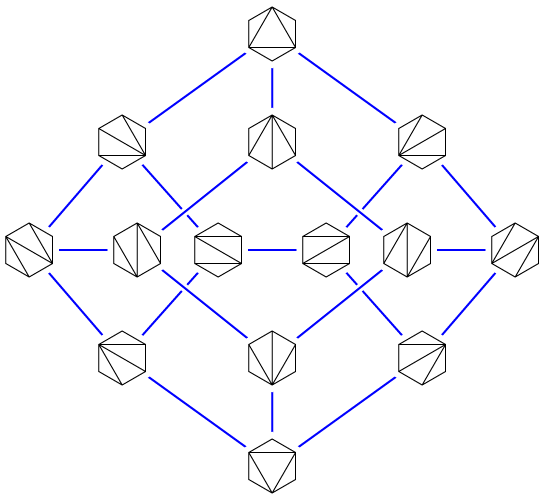My student Daniel Frishberg successfully passed his dissertation defense today!

Several more are on the way but not yet announced. As is typical for our students, the earlier ones involved more of my intervention, the last two were mostly Daniel’s work, and I’m not even likely to be a coauthor on the upcoming ones: the pattern we want to see developing in a new doctorate. The dissertation incorporates the last two of the papers listed above. Most of our students combine three papers to form a dissertation, and the Hanoi graph work would have fit thematically, but just with the two Daniel included it already had plenty of material.

The ICALP reviewers told us that we’ve been underselling one of the results in that paper, on the expansion of the associahedron, so I thought I’d elaborate on that a little more here. An associahedron is a graph whose vertices represent triangulations of a convex polygon (or binary search trees on a given set of keys) and whose edges represent “flips” that remove and replace one diagonal in a triangulation (or that perform a single binary tree rotation). There’s a lot we don’t know about associahedra still, including how to calculate shortest paths (“flip distance”) between vertices efficiently.The version of expansion we’re using is edge expansion,

$\min_{X\subset V(G)}\frac{\vert\partial X\vert}{\min(\vert X\vert,\vert V(G)\setminus X\vert)},$

where $$\partial X$$ represents the set of edges having one endpoint in $$X$$. There are many other graphs of local moves in state spaces that, like the associahedron, can also be given the structure of the vertices and edges of a convex polytope, and in many such cases these have constant expansion. In fact, Milena Mihail and Umesh Vazirani conjectured some time prior to 1992 that every polytope whose vertex coordinates are all 0 or 1 has expansion at least one (for this history see e.g. Kaibel, “On the Expansion of Graphs of 0/1-Polytopes”, arXiv:math/0112146), and it was a big breakthrough in 2018 when Nima Anari, Kuikui Liu, Shayan Oveis Gharan, and Cynthia Vinzant proved it for flip graphs of matroids (arXiv:1811.01816). The associahedra are not 0-1 polytopes, but one can still ask what their expansion is. The ICALP paper gets within a logarithmic factor of the right answer: it proves that the expansion is $$\Omega\bigl(1/(\sqrt n\log n)\bigr)$$ and $$O(1/\sqrt n)$$. The lower bound is the part that fits into the machinery of Daniel’s thesis, but it is the upper bound that the referees told us we were underselling. It is the first time we have seen that the expansion of the associahedron is smaller than a constant.

To prove this upper bound (in appendix C of arXiv:2207.09972), we merely have to find a partition of the space of all triangulations into two subsets with few edges connecting them. This partition is defined very simply, as follows:

• For each triangulation, look at the triangle containing the center point of the polygon.
• This triangle has three sides. Define their “length” combinatorially, as the number of polygon sides they cut off. Thus, the three lengths sum to $$n$$, and the shortest is at most $$n/3$$.
• Put a triangulation into one side of the partition when its central triangle has a very short side, of length less than $$n/6$$, and into the other side of the partition otherwise.

There are always three flips that move one of the vertices of the central triangle, unless the short side is only one edge long. But short motions of these vertices are likelier than long ones, enough so to keep the expansion small. The details involve doing some sums with Catalan numbers.

The next step for Daniel will be to start an assistant professor position at Cal Poly in San Luis Obispo in the fall.

Congratulations, Daniel!